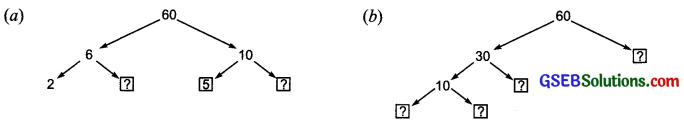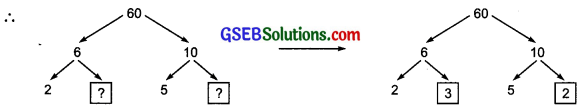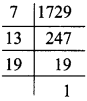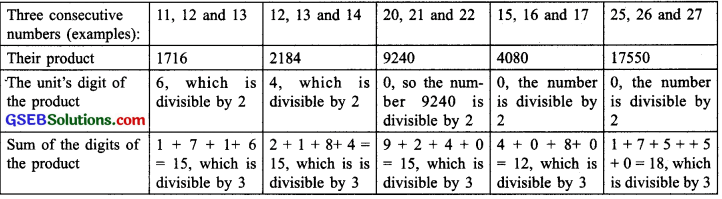# GSEB Solutions Class 6 Maths Chapter 3 Playing With Numbers Ex 3.5

Gujarat Board GSEB Textbook Solutions Class 6 Maths Chapter 3 Playing With Numbers Ex 3.5 Textbook Questions and Answers.

## Gujarat Board Textbook Solutions Class 6 Maths Chapter 3 Playing With Numbers Ex 3.5

Question 1.
Which of the following statements are true?
(a) If a number is divisible by 3, it must be divisible by 9.
(b) If a number is divisible by 9, it must be divisible by 3.
(c) A number is divisible by 18, if it is divisible by both 3 and 6.
(d) If a number is divisible by 9 and 10 both, then it must be divisible by 90.
(e) If two numbers are co-primes, at least one of them must be prime.
(f) All numbers which are divisible by 4, must also be divisible by 8.
(g) All numbers which are divisible by 8, must also be divisible by 4.
(h) If a number exactly divides two numbers separately, it must exactly divide their sum.
(i) If a number exactly divides the sum of two numbers, it must exactly divide the two numbers separately.
Solution:
(a) False statement
(b) True statement
(c) False statement
(d) True statement
(e) False statement
(f) False statement
(g) True statement
(h) True statement
(i) False statementQuestion 2.
Here are two different factor trees for 60. Write the missing numbers.Solution:
(a) 62 x 3 and 10 = 5 x 2(b) 10 = 5 x 2,60 = 30 x 2 and 30 = 10 x 3Question 3.
Which factors are not included in the prime factorization of a composite number?
Solution:
The factor 1 and the number itself are not included in the prime factorization of a composite number.

Question 4.
Write the greatest 4-digit number and express it in terms of its prime factors.
Solution:
The greatest four-digit number = 9999
We have:The prime factorization of 9999
= 3 x 3 x 11 x 101Question 5.
Write the smallest 5-digit number and express it in the form of its prime factors.
Solution:
The smallest 5 – digit number = 10000
We have:The prime factorization of 10000
= 2 x 2 x 2 x 2 x 5 x 5 x 5 x 5Question 6.
Find all the prime factors of 1729 and arrange them in ascending order Now state the relation, if any, between two consecutive prime factors.
Solution:
We have:The prime factorisation of
1729 = 7 x 13 x 19
Now, ascending order of the prime factors of 1729 is: 7, 13, 19
Now, we have:
19 – 13 = 6
13 – 7 = 6
The relation is: the difference of two consecutive prime factors is 6.Question 7.
The product of three consecutive numbers is always divisible by 6. Verify this statement with the help of some examples.
Solution:
Let us consider the following examples:In each case the product is divisible by both 2 and 3.
They are also divisible by 6.
Thus, it is verified that the product of three consecutive numbers is always divisible by 6.Question 8.
The sum of two consecutive odd numbers is divisible by 4. Verify this statement with the help of some examples.
Solution:
Let us consider the following examples.Since, each of the numbers formed from the last two digits is divisible by 4, so the numbers (sums) are also divisible by 4.
Thus, the sum of two consecutive odd numbers is always divisible by 4.

Question 9.
In which of the following expressions, prime factorization has been done?
(a) 24 = 2 x 3 x 4
(b) 56 = 7 x 2 x 2 x 2
(c) 70 = 2 x 5 x 7
(d)54 = 2 x 3 x 9
Solution:
(a) 24 = 2 x 3 x 4 : since; 4 is not a prime number,
24 = 2 x 3 x 4 is not a prime factorisation.

(b) 56 = 7 x 2 x 2 x 2: Since, all the factors are prime numbers,
It is a prime factorisation.

(c) 70 = 2 x 5 x 7: Since all the factors are prime numbers, it is a prime factorisation.

(d) 54 = 2 x 3 x 9: Since, 9 is not a prime number,
54 = 2 x 3 x 9 is not a prime factorisation.Question 10.
Determine if 25110 is divisible by 45.
Hint: 5 and 9 are co-prime numbers. Test the divisibility of the number by 5 and 9.
Solution:
45 = 5 x 9, where 5 and 9 are co-primes.
To check the divisibility by 5 and 9, we have that
25110 is divisible by 5, because its unit’s digit is 0.
Again, the sum of the digits = 2 + 5 + 1 + 1 + 0 = 9
25110 is divisible by 9.
Since, 25110 is divisible by both 5 and 9 and, 5 and 9 are co-primes,
25110 is divisible by 9 x 5.
i.e. 25110 is divisible by 45.Question 11.
18 is divisible by both 2 and 3. It is also divisible by 2 x 3 = 6. Similarly, a number is divisible by both 4 and 6. Can we say that the number must also be divisible by 4 x 6 = 24? If not, give an example to justify your answer.
Solution:
4 and 6 are not co-prime numbers.
It is not necessary that a number divisible by both 4 and 6 will be divisible by their product 4 x 6 (= 24).
Example: 36 and 60 are divisible by both 4 and 6 but not by 24.

Question 12.
I am the smallest number, having four different prime factors. Can you find me?
Solution:
Since, the smallest four prime numbers are: 2, 3, 5 and 7
We have : 2 x 3 x 5 x 7 = 210
The required number = 210